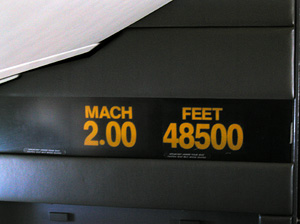A# Mach numberConcorde Mach speed indicator.

Mach number is a unit of speed, named after the Austrian physicist Ernst Mach, equal to the ratio of the speed of a moving object to the speed of sound in the surrounding medium under ambient conditions.

The actual speed of sound varies depending on the altitude above sea level because sound travels at slightly different speeds at different temperatures, and the temperature varies according to altitude. At sea level, the speed of sound, known as Mach 1.0, is about 761 miles per hour (1,225 kilometers per hour). At 20,000 feet (6,096 meters), the speed of sound is 660 mph (1,062 kilometers per hour).

If an aircraft is traveling at half the speed of sound, it is said to be traveling at Mach 0.5. A speed of Mach 2.0 is twice the speed of sound. Because the speed of sound varies, a particular speed at sea level expressed as a Mach number would be faster than the same speed at 30,000 feet (9,144 meters), which would be faster than the same speed at 40,000 feet (12,192 meters). In other words, Mach 2 at sea level is a greater number of miles per hour (or kilometers per hour) than Mach 2 at 30,000 feet, which is a greater number of miles per hour than Mach 2 at 40,000 feet. When an aircraft reaches Mach 1, it is said to 'break the sound barrier.'

The following ranges of Mach numbers, known as regimes of flight, have been generally accepted to classify speeds:

 M < 0.8 subsonic 0.8 < M < 1.2 transonic 1.2 < M < 5.0 supersonic M > 5.0 hypersonic

A critical Mach number is the speed of an aircraft (below Mach 1) when the air flowing over some area of the airfoil has reached the speed of sound. For instance, if the air flowing over a wing reaches Mach 1 when the wing is only moving at Mach 0.8, then the wing's critical Mach number is 0.8.

## Variation of Mach number

The Mach number depends on the speed of sound in the gas and the speed of sound depends on the type of gas and the temperature of the gas. The speed of sound varies from planet to planet. On Earth, the atmosphere is composed of mostly diatomic nitrogen and oxygen, and the temperature depends on the altitude in a rather complex way. Scientists and engineers have created a mathematical model of the atmosphere to help them account for the changing effects of temperature with altitude. Mars also has an atmosphere composed of mostly carbon dioxide. There is a similar mathematical model of the martian atmosphere. We have created an atmospheric calculator to let you study the variation of sound speed with planet and altitude.